Click to Chat

1800-1023-196

+91-120-4616500

CART 0

• 0

MY CART (5)

Use Coupon: CART20 and get 20% off on all online Study Material

ITEM
DETAILS
MRP
DISCOUNT
FINAL PRICE
Total Price: Rs.

There are no items in this cart.
Continue Shopping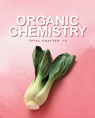```Solved Problems on Biomolecules

Subjective

Question 1: In E. coli DNA, the AT/GC ratio is 0.93. If the number of moles of adenine in the DNA sample is 558,000, calculate the number of moles of guanine present.Solution: Number of moles of adenine must be equal to that of thymine

(A + T) = 558,000 + 558,000 = 1116000

Since (A + T)/(C + G ) = 0.93
Therefore, no of moles of C + G

C + G = 1116000/0.93 = 1200000

Since number of moles of C = number of moles of G
Number of moles of guanine =1200000/2 = 600000

Question 2:  How will you synthesize alanine from acetylene?
Solution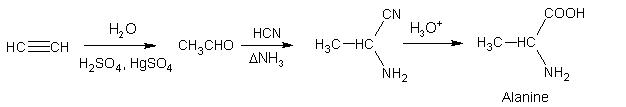Question 3: Write the structure of alanine at pH =2 and pH = 10.
Solution. Amino acids exist as zwitter ions (I) in aqueous solution. In presence of acids (pH=2) basic COO– accepts a proton to give cation (II) but in presence of base (pH = 10), the acidic   donates a proton to the base and thus exists as anion (III).Question 4: Glycine exists as (H3N+CH2COO–) while anthranilic acid (P–NH2C6H4– COOH) does not exist as dipolar ions.
Solution.– COOH is too weakly acidic to transfer H+ to the weakly basic – NH2 attached to the electron withdrawing benzene ring. When attached to an aliphatic carbon, the – NH2 is sufficiently basic to accept H+ from – COOH group

Question 5: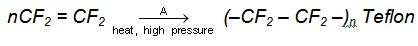Solution:  A = (NH4)2S2O8

Question 6: Complete the following reaction: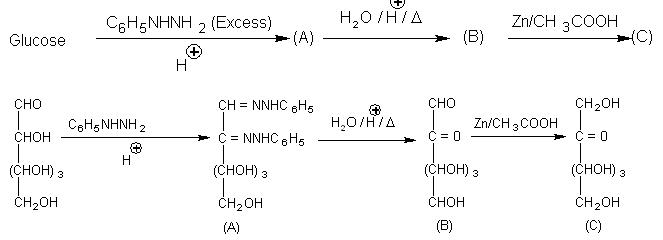Question 7: In the structure of aspartame (an artificial sweetner) which is a peptide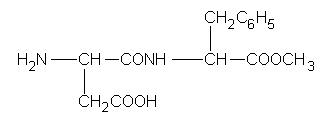(i)  Identify the four functional groups
(ii) Write the zwitterionic structure
(iii) Write the structure of amino acids obtained from the hydrolysis of aspartame
Solution:

(i) Four functional groups in aspartame are
– NH2 (amino group)
– COOH (carboxyl group)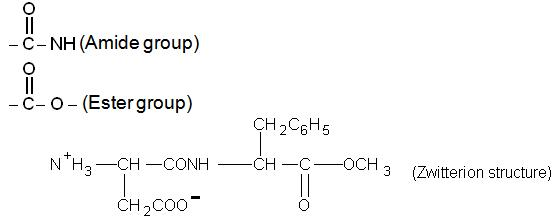(iii) Hydrolysis of aspartame gives following two amino acids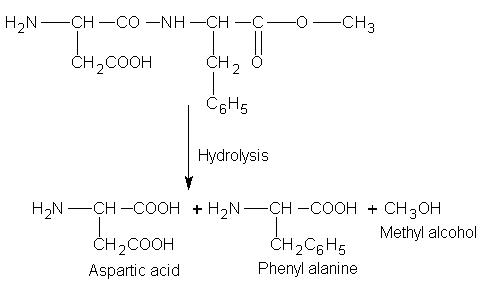Question 8: Following two amino acids lysine and glutamine form dipeptide linkage. What are two possible dipeptide?

Solution: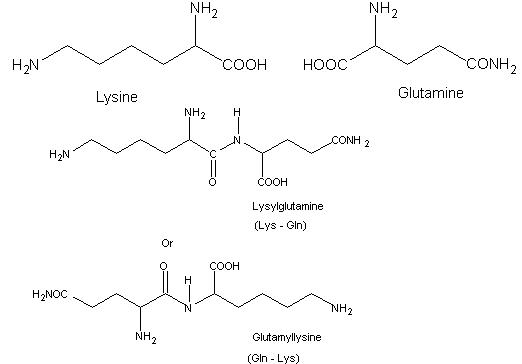Question 9: When primary bromides are treated with a solution of NaI in pure acetone precipitate generates within 3 minutes at 25°C whereas primary chlorides when treated with same reagent precipitate generates within 6 minutes at 50°C. Explain.
Solution: The reaction is probably of the SN2 type involving a bimolecular attack of the iodide ion upon the carbon atom carrying the chloride or bromine. Now Br– is better leaving group when Cl– (as it is a weaker base). So the rate of the reaction for bromides is faster.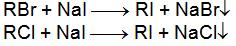Question 10: Glucose and fructose give the same osazone? Explain
Solution. During osazone formation reaction occurs only at C1and C2 while rest of the molecule remains same, and glucose & fructose differ from each other only in arrangement of atoms at C1 and C2, so they give same osazone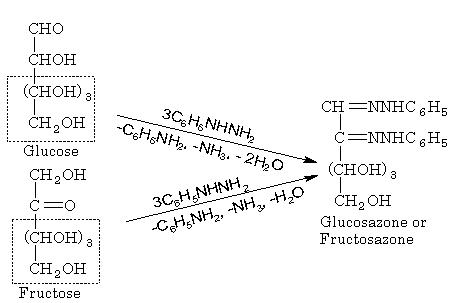Question 11: pecific rotations of  -anomer of given mono saccharide is + 112° and for
-anomer is + 19°. Specific rotation of equilibrium mixture is 52.7° calculate % composition of  α and β anomers in the equilibrium mixture.

Solution: Suppose fraction of  and anomers is X and Y respectively

X + Y = 1 ……………………..(1)
112X + 19Y = 52.7……………(2)
By solving above two equations
X = 36.2%,   Y = 63.8%

Question 12: Predict the products of the treatment of glycine with
(a) Aqueous NaOH
(b) Aqueous HCl
(c) Acetic anhydride
(d) NaNO2 + HCl

Solution.
(a) H2N.CH2COO–Na+
(b) Cl– + H3N+CH2COOHObjective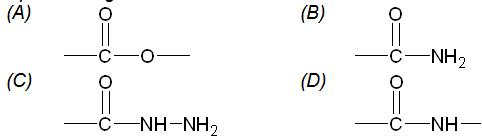Solution.(D). Peptide linkage is formed when two amino acid combine with elimination of water.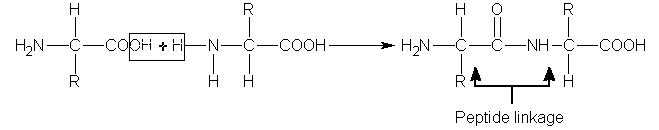Question 2. If one strand of a DNA has the sequence -AATCGTAGGCAC-.What is the sequence of complementary strand.
(A)    TTAGCATCCGTG    (B)    TTAGCATCCGTG
(C)    UUTCGTAGGCAC    (D)    UUAGCAUCCGUG
Solution. DNA is AATCGTAGGCAC its complementary strand will be TTAGCATCCGTG.     Hence (B) is correct answer.

Question 3. Structure of DNA molecule is
(A)    single stranded     (B)    linear
(C)    branched              (D)    double strand
Solution. DNA always exists as double stranded, only at the time of replication or transcription it acts as template (single strand) for synthesis of new strand.

Question 4. Match items in list –I with those in the list – II form the combinations shown

List – I

List – II

(i) Pepsin

(A) Genetic material

(ii) Nucleic acid

(B) Digestive enzyme

(iii) Ascorbic acid

(C) Antibiotic

(iv) Testosterone

(D) Sex hormone

(E) Vitamin

(A)    I – B; II – A ; III – C ; IV – E     (B)    I – A ; II – B ; III – E ; IV – C
(C)    I – B ; II – A ; III – E ; IV – D     (D)    I – C ; II – A ; III – A ; IV – D
Solution.  Answer is (B), pepsin is a diagestic enzyme, nucleic acid is genetic material, ascorbic acid is vitamin C and testosterone is sex hormone.

Question 6. Which substance is not present in nucleic acids?
(A)    cytosine
(C)    thymine
(D)    guanidine
Solution.  Nitrogenous base in nucleic acids are
Pyrimidine – Cytosine and Thymine or uracil

Question 7.    Nylon-66 is a polyamide of
(A)    Vinylchloride and formaldehyde
(B)    Adipic acid and methyl amine
(C)    Adipic acid and hexamethylene diamine
(D)    Formaldehyde and melamine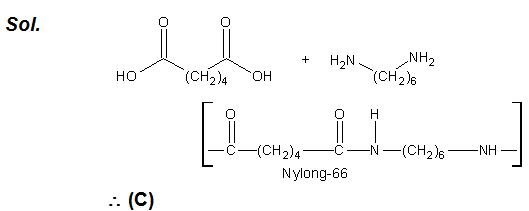Question 8 .    Which of the following is not a condensation polymer?
(A)    Glyptal
(B)    Nylon-66
(C)    Dacron
(D)    PTFE
Solution.    Others are condensed polymer(C)

Question 9.    Which of the following is an example of basic dye?
(A) Alizarine
(B) Indigo
(C) Malachite
(D) Orange – I

Solution: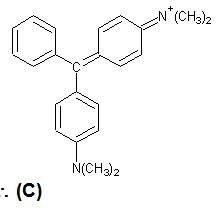Question 10. Which one of the following is a copolymer?
(A)    Buna-S
(B)    Polyvinyl chloride
(C)    Polypropylene
(D)    Poly-cis-isoprene

Solution (A). Polymers whose repeating units are derived from two or more types of monomers units, are called copolymer. Only Buna-S is obtained by the copolymerization of butadiene and styrene monomers. Other polymers (B), (C) and (D) are homopolymers, because they consists of only one type of monomers.

True and False

Question 11.Essential amino acids are synthesized inside the body.
Solution. False

Question 12:  Amino acids are linked together by glycoside bond.
Solution: False

Question 13. Sequence one strand DNA has AATCGAGGAC and complementary sequence is TTAGCATCCGTG.
Solution: True

Question14: Glycogen is a storage food in animal.
Solution: True

Question 15.    At isoelectric point migration of amino acid is stopped.
Solution: True

You can also refer to the following related links

JEE Organic Chemistry Syllabus

Reference books of Organic Chemistry

To read more, Buy study materials of Biomolecules comprising study notes, revision notes, video lectures, previous year solved questions etc. Also browse for more study materials on Chemistry here.
```### Course Features

• 731 Video Lectures
• Revision Notes
• Previous Year Papers
• Mind Map
• Study Planner
• NCERT Solutions
• Discussion Forum
• Test paper with Video Solution